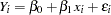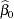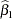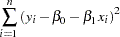Introduction to Statistical Modeling with SAS/STAT Software
 Classical Estimation Principles

An estimation principle captures the set of rules and procedures by which parameter estimates are derived. When an estimation principle "meets" a statistical model, the result is an estimation problem, the solution of which are the parameter estimates. For example, if you apply the estimation principle of least squares to the SLR model, the estimation problem is to find those valuesandthat minimizeThe solutions are the least squares estimators.

The two most important classes of estimation principles in statistical modeling are the least squares principle and the likelihood principle. All principles have in common that they provide a metric by which you measure the distance between the data and the model. They differ in the nature of the metric; least squares relies on a geometric measure of distance, while likelihood inference is based on a distance that measures plausability.Previous Page | Next Page | Top of Page